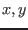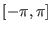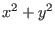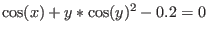Next: Integration Up: ALIAS-Maple Previous: Simplification procedures

• La page de J-P. Merlet
• La page Présentation de HEPHAISTOS
• La page "Présentation" de l'INRIA

Subsections

# Introduction

You may solve an optimization problem with the ALIAS library by using one of the following procedures:



.

Note that these procedures are only special variants of the general solving procedures of ALIAS-Maple. Hence most of the parameters setting for these procedures are also valid for the optimization procedures. Specific parameters for these procedure are presented in section 5.3.

In the list of functions that are provided to these procedures the last one must be the function whose extremum are looked for, while the other one are supposed to be constraints. The interval solution that are returned will verify:

• for the constraints:
• for inequality constraint: for any value of the unknowns within the solution ranges the inequality is strictly satisfied
• for equality constraint: if the width of the interval solution is less than ALIAS/epsilon then the interval evaluation of the constraint include 0, while if this is not the case then the width of the interval evaluation of the function include 0 and its width is less than ALIAS/fepsilon
• for the function that is to be minimized or maximized: the real minimum or maximum of the function is at a distance at most ALIAS/eepsilon (default value: 1e-6) from the result provided by ALIAS

You may also us the gradient of the function to be minimize or maximized to improve the efficiency of the procedure. The MinimizeGradient and MaximizeGradient procedures may be used for this purpose and have the same argument than the Minimize and Maximize procedure.

There are also procedures to compute at the same time the minimum and maximum values of a function, eventually under constraint. The Minmax and MinMaxGradient procedures may be used for this purpose. The only difference with the previous procedures is that the arguments should be ended by Min, Max which provide the minimum and maximum of the function (being understood that the function that is considered is always the last in the list, the other one being the constraints).

However in that case a global variable of the ALIAS Maple library may play an important role:
ALIAS/stop_minmax. Its default value is set to 0 but:

• if set to 1: the procedure will search the minimal and maximal value of the last function in the list but will exit if at some point it is shown that the minimum and maximum value have opposite sign. If this is not the case the procedure will still provide the minimal and maximal value of the function up to the given accuracy
• if set to 2: here the main purpose is to determine if the minimum and maximum of the function have the same sign. None of the Min, Max value will be significant from an optimum viewpoint: they are used only to indicate the sign of the extremum.

The flag allow_storage may also be of interest: if set to 1 (default value: 0) the current estimation of the optimum is written in the file .opti in a sequential way.

All these procedures accept optionally as least argument a string Simp that indicates the name of a simplification procedure (see chapter 4) whose C++ code is available in the file Simp.C. Among the simplification procedures provided by ALIAS-Maple the use of the HullIConsistency procedure with the key-word Optimum may be interest for an optimization problem, see section 4.2.1.

You may also specify initial value for the minimum or maximum value of the optimized function by setting ALIAS/opt_min, ALIAS/opt_max.

# Examples

Let consider the following example:


Minimize([cos(x)+y*cos(y)^2-0.2,x^2+y^2],[x,y],[[-Pi,Pi],[-Pi,Pi]],Min);

will return the value ofin the rangewhich minimize the value ofunder the constraintand Min will contain the minimal value. In the previous example we will get the following result:

>Minimize([cos(x)+y*cos(y)^2-0.2,x^2+y^2],[x,y],[[-Pi,Pi],[-Pi,Pi]],Min);
[[[-.957582, -.957582], [-.474626, -.474626]],

[[-.957582, -.957582], [-.474626, -.474626]]]
>Min;
[[1.14223, 1.14223]]

You may notice that Min is a range and that Minimize returns two solutions for x,y. This is so because Minimize may deal with function having interval coefficients: hence the procedure will return a range for the minimal value of the function and the values of x,y at which the lower and upper bound have been obtained. Hence we may change the previous example with:

Minimize([cos(x)+y*cos(y)^2-0.2,(x+INTERVAL(0..0.01))^2+y^2],[x,y],[[-Pi,Pi],[-Pi,Pi]],Min);
[[[-.958271, -.958271], [-.473241, -.473241]],

[[-.957582, -.957582], [-.474626, -.474626]]]

> Min;
[[1.12307, 1.14233]]

Note that for this example we may have generated a simplification procedure with:

HullIConsistency([x^2+y^2-(Optimum-ALIAS/eepsilon)<=0],[x,y],0,"Simp");
Minimize([cos(x)+y*cos(y)^2-0.2,x^2+y^2],[x,y],[[-Pi,Pi],[-Pi,Pi]],Min,"Simp");

which allows to decrease almost by half the computation time.

# Specific parameters for the optimization procedures

We indicate here parameters that appear in the optimization procedures, their meaning, their default value and the corresponding name in the C++ library.

 Parameter name Meaning C++ equivalent ALIAS/eepsilon Accuracy on the optimum ALIAS/optimize type of optimization ALIAS_Optimize (automatically set by the procedure) ALIAS/optimize_lo_a threshold on the distance between ALIAS_LO_A the current minimum and a new minimum to call the local optimizer ALIAS/optimize_lo_b threshold on the distance between ALIAS_LO_B the current maximum and a new maximum to call the local optimizer ALIAS/stop_minmax allows to exit from the ALIAS_Stop_Sign_Extremum algorithm if the optimum have opposite sign ALIAS/stop_opt_sol allows to exit from the ALIAS_Stop algorithm if the optimum is better than a threshold ALIAS/opt_sol_min threshold for stop_opt_sol ALIAS_Extremum ALIAS/opt_sol_max threshold for stop_opt_sol ALIAS_Extremum ALIAS/opt_max,ALIAS/opt_min` allows to specify initial values for the minimum and/or maximumNext: Integration Up: ALIAS-Maple Previous: Simplification procedures